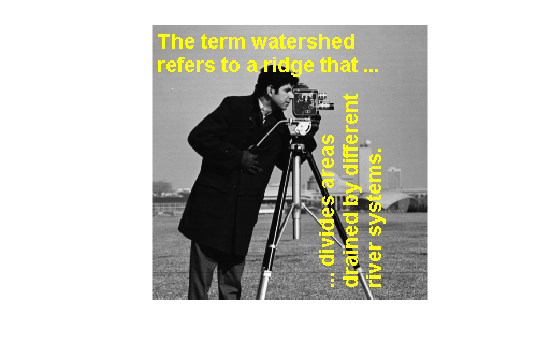# imoverlay

Burn binary mask into 2-D image

## Syntax

``B = imoverlay(A,BW)``
``B = imoverlay(___,color)``

## Description

example

````B = imoverlay(A,BW)` fills the grayscale or RGB input image, `A`, with a solid color where the input binary mask, `BW`, is `true`.```
````B = imoverlay(___,color)` lets you specify the color that `imoverlay` uses to fill the image. `color` is a valid MATLAB® color specification.```

## Examples

collapse all

Read grayscale image into the workspace.

`A = imread('cameraman.tif');`

Read binary image into the workspace.

`BW = imread('text.png');`

Burn the binary image into the grayscale image, choosing the color to be used.

`B = imoverlay(A,BW,'yellow');`

Display the result.

```figure imshow(B)```## Input Arguments

collapse all

Input image, specified as a real, nonsparse 2-D matrix.

Data Types: `single` | `double` | `int16` | `uint8` | `uint16` | `logical`

Mask image, specified 2-D logical matrix the same size as `A`.

Data Types: `single` | `double` | `int16` | `uint8` | `uint16` | `logical`

Color used for the overlay, specified as a MATLAB color specification. For example, if you want to specify the color red, you could use any of the following specifications: `'red'`,`'r'`, or `[1 0 0]`.

## Output Arguments

collapse all

Output image, returned as a 2-D matrix of class `uint8`.